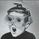Dilihat 1108
1108
Measures the period's change in terms of the instrument's value (e.g. pip, dollar, etc) instead of as a percentage. I generally use it on a daily time frame with a period=1 to see how the current day's move compares with prior days' moves in order to gain a perspective into how this move ranks historically.
```study(title="Rate Of Change", shorttitle="ROC")
source = close, length = input(9, minval=1)
roc = (source - source[length])
plot(roc, color=blue, title="ROC")
hline(0, title="Zero Line")
```Hello Can you look at making a ROC of the 20 SMA like this. See video one hour in: https://youtu.be/I_aqnns0vJ0
Jawab